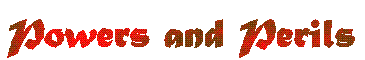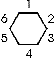# The Coordinate System

The coordinates in the Perilous Lands section can be given in two ways. The first is "aa-bbcc", where "aa" is the map number, "bb" is the hex column starting from the left (01 means first column on page) and cc is the hex row starting from the bottom (01 means bottom-most hex).The second method is to give a relative coordinate. This is often used when a location is near to a named city (within three hexes). In this case coordinates are given of the form of "name-path", where "name" is the name of the object and the "path" is 1-3 directions to travel to get to that point. In this system, due north is "1", NE is "2", SE is "3" due south is "4", SW is "5" and NW is "6". Thus, the fort nearest to Matan is located at 8-0112, or could be noted as "Matan-555"